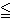The Assignment ProblemSuppose you are the owner of a company and need to delegate tasks to your employees. You’ve generated a table that tells how long (in minutes) you think it would take each person to accomplish each individual task (called Jobs). Your goal is to find an assignment of people to jobs that minimizes the total amount of time it will take to complete all jobs. The requirements are that each job must be completed by only one person, and each person can complete only one job.

We can think of the employees at the job as our supply and the tasks as the demand. In order for this problem to have a feasible solution, we must have enough people (supply) to complete the number of jobs (demand). Because of this, our examples will all include situations where there are exactly the same number of people as jobs.

To solve this problem, we must first generate an initial assignment and see how good this assignment is. There are several ways of generating an initial solution, but two that I wanted to focus on are the “NorthWest Corner Rule” and the “Minimum Matrix Method”.

1. The Northwest Corner Rule considers the matrix and repeatedly assigns the top remaining row to the left-most remaining column. If we think of the cost matrix as a being like a map then “top” becomes similar to “north” and left-most becomes similar to “west”, hence the derivation of the name. In assignment problems, this will result in the main diagonal being selected.
2. The Minimum Matrix Method is an iterative method that searches the matrix for the minimum cell in the matrix and assigns that person to the associated job and removes them from consideration and repeats itself until all people have been assigned to jobs.

Once we have formulated an initial feasible solution, we need to check it for optimality. To do this, we use the Network Simplex Method, where we build a basis based on this initial solution. When we consider this problem as a network flow problem, a basis for the problem is a spanning tree (one less than twice the number of nodes in the graph that does not have any cycles) of the network. Because the assignment solution only contains one edge for every two nodes in the graph, we need to add a number of edges to the basis that contains no flow (which makes the solution degenerate) to form this spanning tree.

Once a spanning tree is formulated, we can solve for the dual variables by arbitrarily setting one node’s dual value to zero and solving for the remaining dual variables under the requirement that all arcs in the basis (spanning tree) must satisfy the equation uk + vi = cki for each person k and job i.

When we have dual variables, we can check to see if this solution is optimal by checking to see if all the other constraints are violated. This means that for every person k and every job i, we must have uk + vicki (notice that this is a more relaxed version of what we had when we were solving for the dual variables themselves).

If a constraint is found to be violated, then we need to add the associated edge to the basis and remove an edge on the cycle that is formulated as a result, which generates a new solution.

So check out The Assignment Problem Script and let me know what you think.

Similar Posts:

This site uses Akismet to reduce spam. Learn how your comment data is processed.